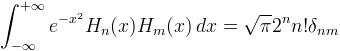﻿OrthogonalPolynomials.HermiteH MethodComputes the value of a (physicists') Hermite polynomial.

Namespace:  Meta.Numerics.Functions
Assembly:  Meta.Numerics (in Meta.Numerics.dll) Version: 4.1.4Syntax
```public static double HermiteH(
int n,
double x
)```

#### Parameters

n
Type: SystemInt32
The order, which must be non-negative.
x
Type: SystemDouble
The argument.

#### Return Value

Type: Double
The value Hn(x).Exceptions
ExceptionCondition
ArgumentOutOfRangeExceptionn is negative.Remarks

Hermite polynomials are orthogonal on the interval (-∞,+∞) with the weight e-x2.They appear in the solution of the one-dimensional, quantum mechanical, harmonic oscillator.

Statisticans' Hermite polynomials (see HermiteHe(Int32, Double)) are related to physicists' Hermite polynomials via Hn(x) = 2nHn(x √2). Statisticians' Hermite polynomials do not grow as quickly as physicists', and may therefore be preferable for large values of n and x which could overflow Double.See Also